# Class 11 RD Sharma Solutions – Chapter 6 Graphs of Trigonometric Functions – Exercise 6.3

• Last Updated : 08 May, 2021

### Question 1: y = sin2 x

Solution:

As we know that,

Attention reader! All those who say programming isn't for kids, just haven't met the right mentors yet. Join the  Demo Class for First Step to Coding Coursespecifically designed for students of class 8 to 12.

The students will get to learn more about the world of programming in these free classes which will definitely help them in making a wise career choice in the future.

y = sin2 x =On shifting the origin at (0, 1/2), we get

X = x and Y =On substituting these values, we getThe maximum and minimum values of Y areandrespectively and shift it by 1/2 to the up.

As the equation in the form of y = – f(x), the graph become inverted of y = f(x)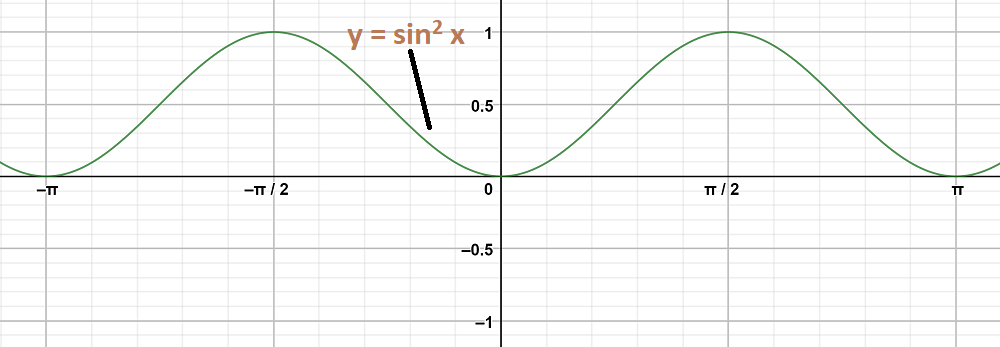### Question 2: y = cos2 x

Solution:

As we know that,

y = cos2 x =On shifting the origin at, we get

X = x and Y =On substituting these values, we getThe maximum and minimum values of Y areandrespectively and shift it by 1/2 to the up.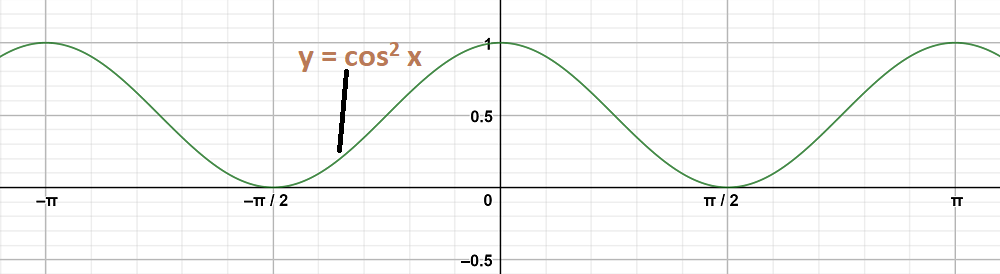### Question 3: y = sin2Solution:

To obtain this graph y-0 = sin2On shifting the origin at (,0), we get

X =and Y = y – 0

On substituting these values, we get

Y = sin2 X

First we draw the graph of Y = sin2 X and shift it by π/4 to the right.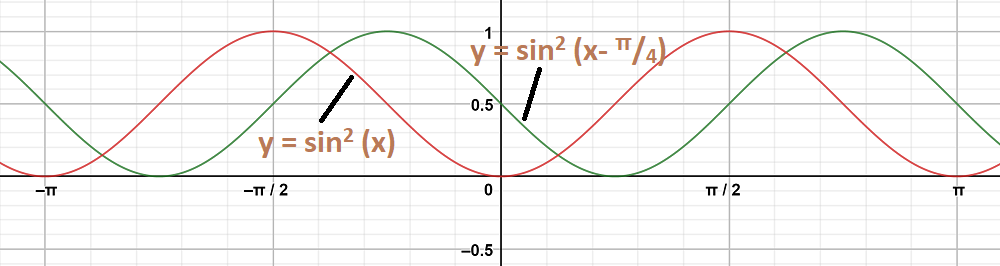### Question 4: y = tan 2x

Solution:

To obtain this graph y = tan 2x,

First we draw the graph of y = tan x and then divide the x-coordinates of the points where it crosses x-axis by 2.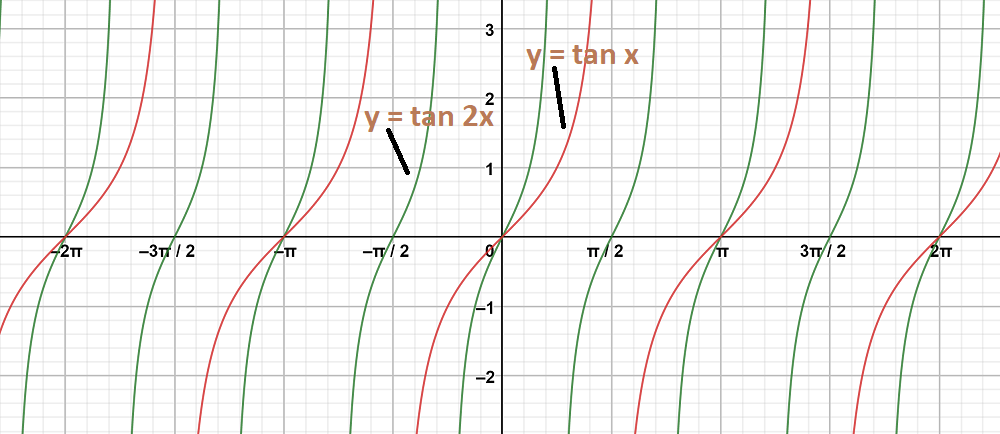### Question 5: y = 2 tan 3x

Solution:

To obtain this graph y = 2 tan 3x,

First we draw the graph of y = tan x and then divide the x-coordinates of the points where it crosses x-axis by 3.

Stretch the graph vertically by the factor of 2.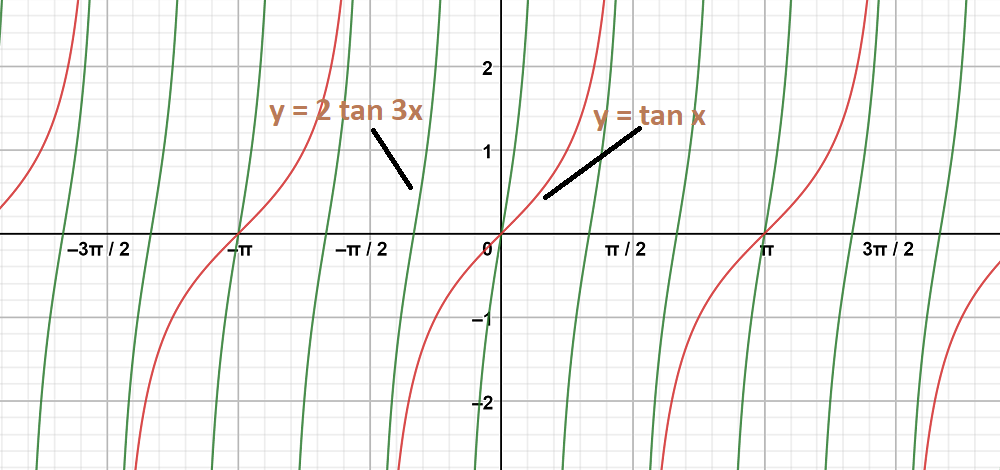### Question 6: y = 2 cot 2x

Solution:

To obtain this graph y = 2 cot 2x,

First we draw the graph of y = cot x and then divide the x-coordinates of the points where it crosses x-axis by 2.

Stretch the graph vertically by the factor of 2.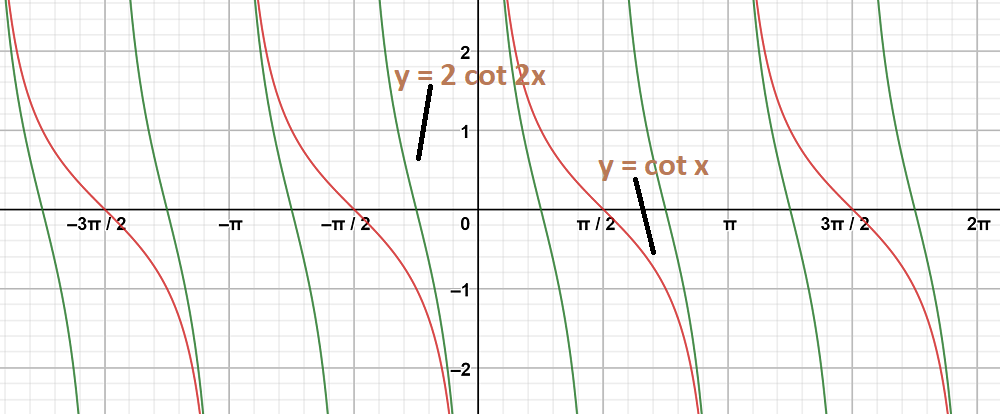### Question 7: y = cos 2x, y = cosSolution:

Graph 1:

y = cos2 x

As we know that,

y = cos2 x =On shifting the origin at (0, 1/2), we get

X = x and Y =On substituting these values, we getThe maximum and minimum values of Y areandrespectively and shift it by 1/2 to the up.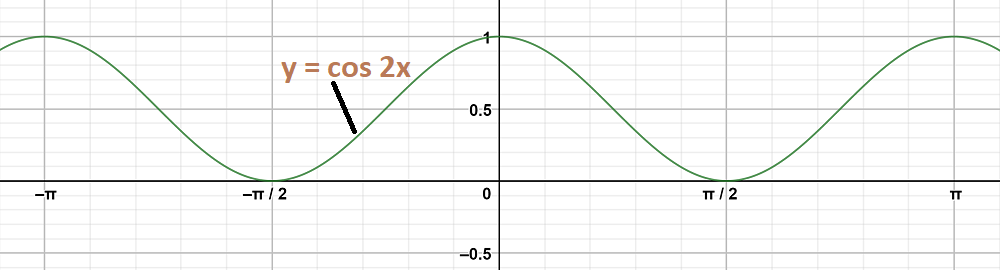Graph 2:

To obtain this graph y-0 = cos (2x-) = cos 2(x-)

On shifting the origin at (π/6, 0), we get

X =and Y = y – 0

On substituting these values, we get

Y = cos 2X

First we draw the graph of Y = cos 2X and shift it by π/6 to the right.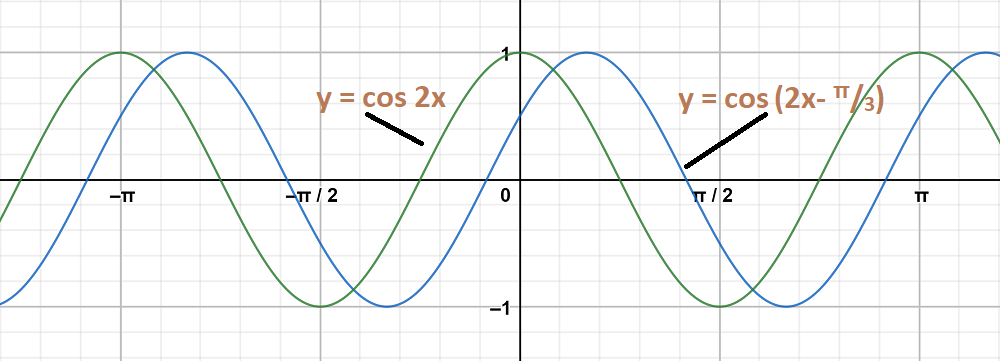The graph y = cos2 x and y = cosare on same axes are as follows: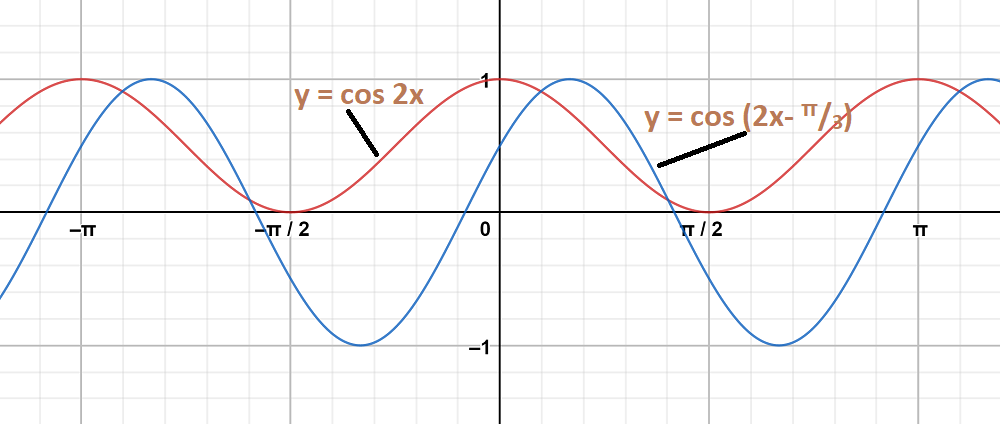### Question 8: y = sin2 x, y = sin x

Solution:

Graph 1:

y = sin2 x

As we know that,

y = sin2 x =On shifting the origin at (0,), we get

X = x and Y =On substituting these values, we getThe maximum and minimum values of Y areandrespectively and shift it by 1/2 to the up.

As the equation in the form of y = – f(x), the graph become inverted of y = f(x)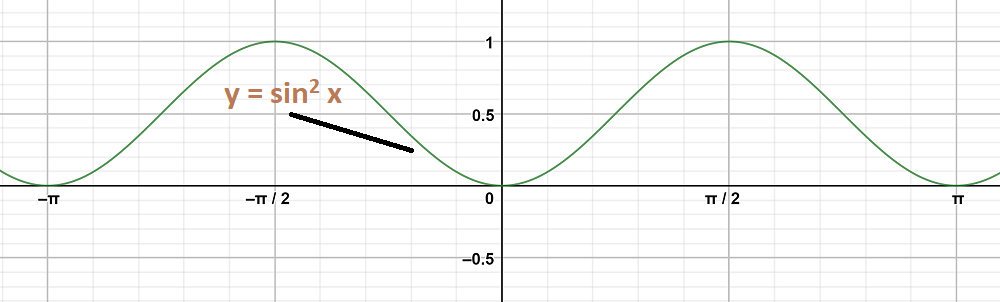Graph 2:

y = sin x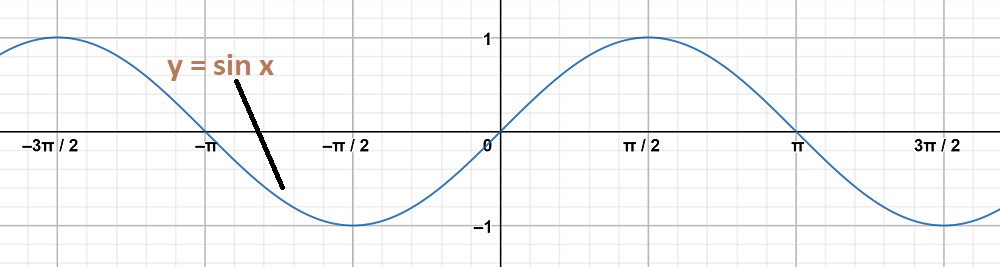The graph y = sin2 x and y = sin x are on same axes are as follows: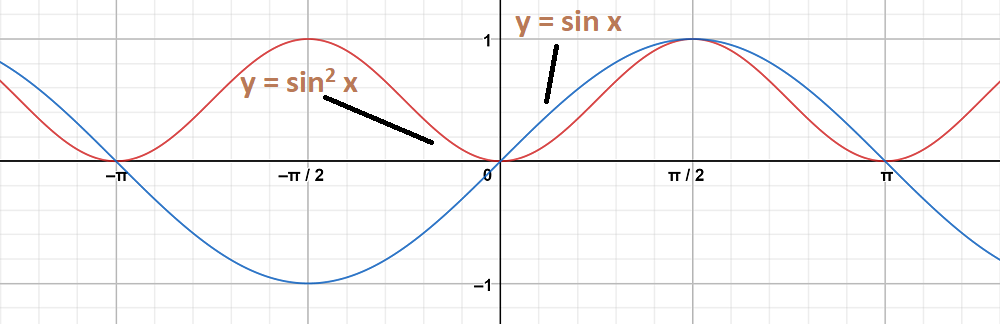### Question 9: y = tan x, y = tan 2x

Solution:

Graph 1:

y = tan x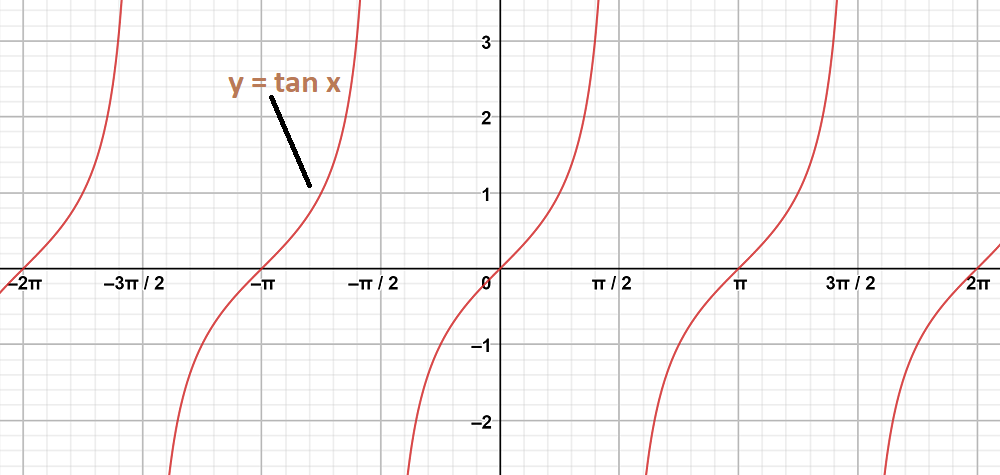Graph 2:

y = tan2 x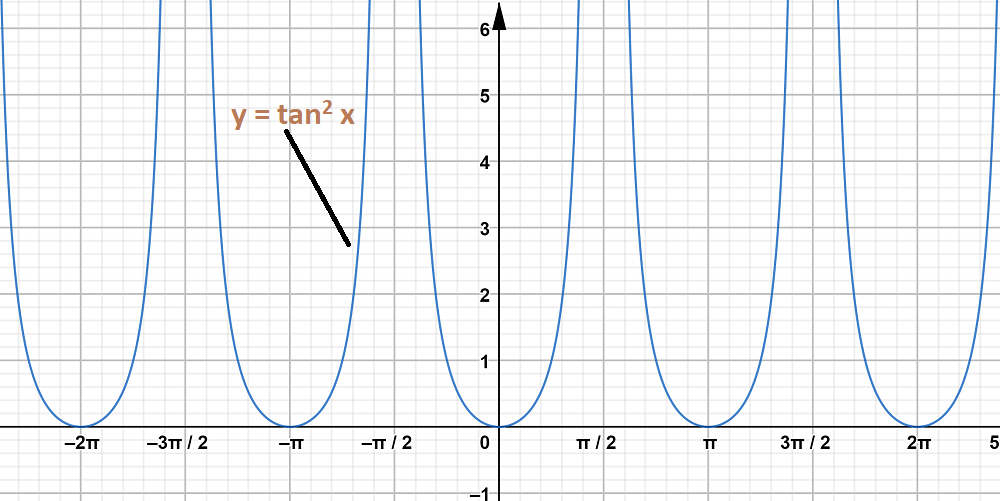The graph y = tan x and y = tan2 x are on same axes are as follows: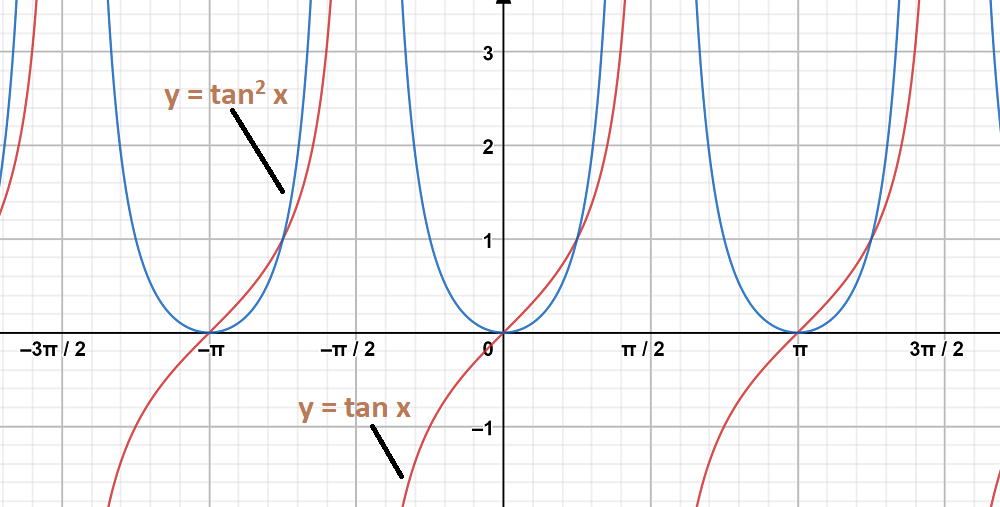### Question 10: y = tan 2x, y = tan x

Solution:

Graph 1:

To obtain this graph y = tan 2x,

First we draw the graph of y = tan x and then divide the x-coordinates of the points where it crosses x-axis by 2.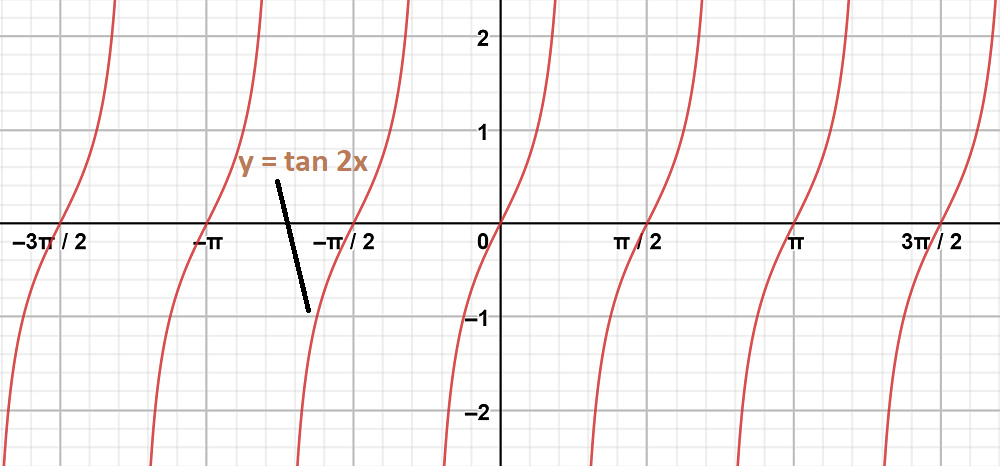Graph 2:

y = tan x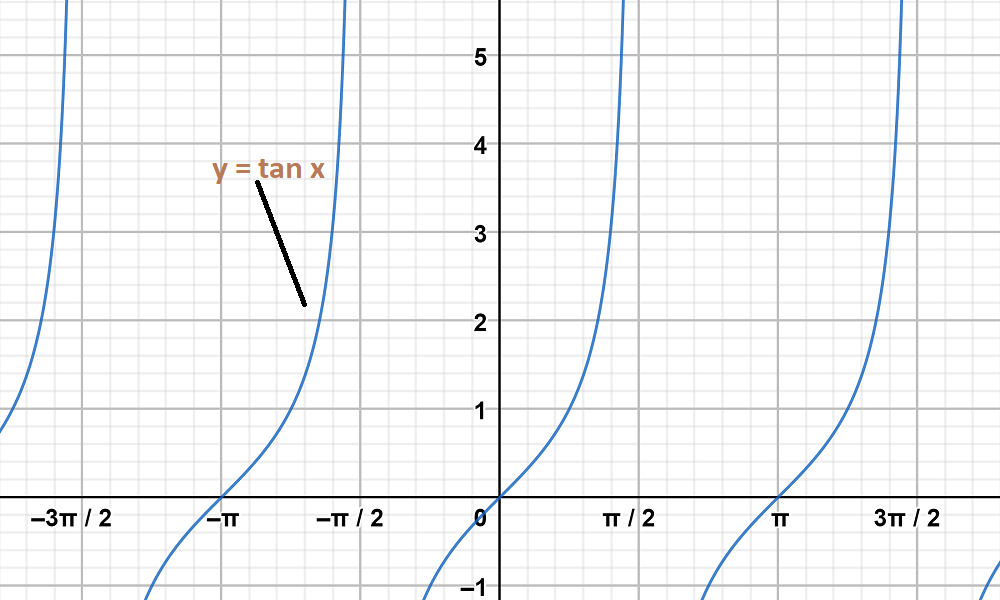The graph y = tan 2x and y = tan x are on same axes are as follows: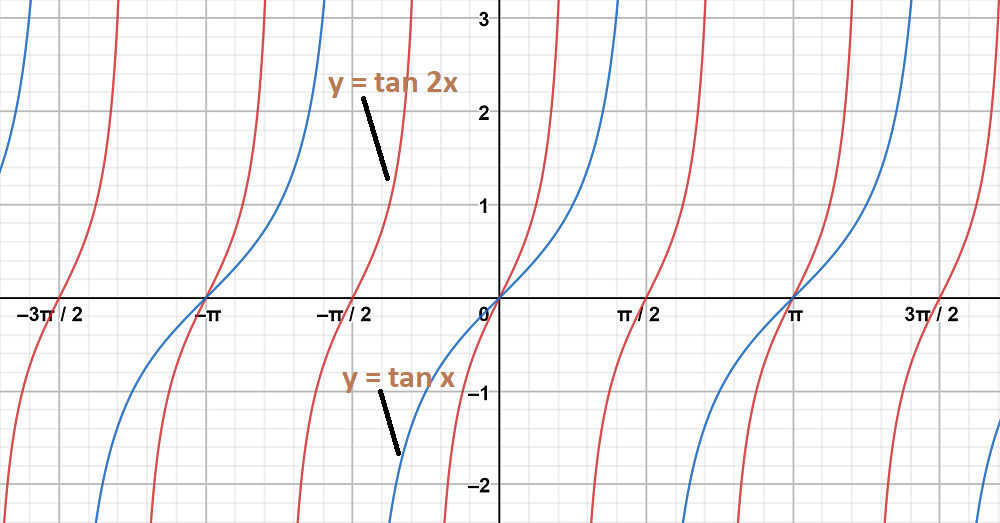My Personal Notes arrow_drop_up